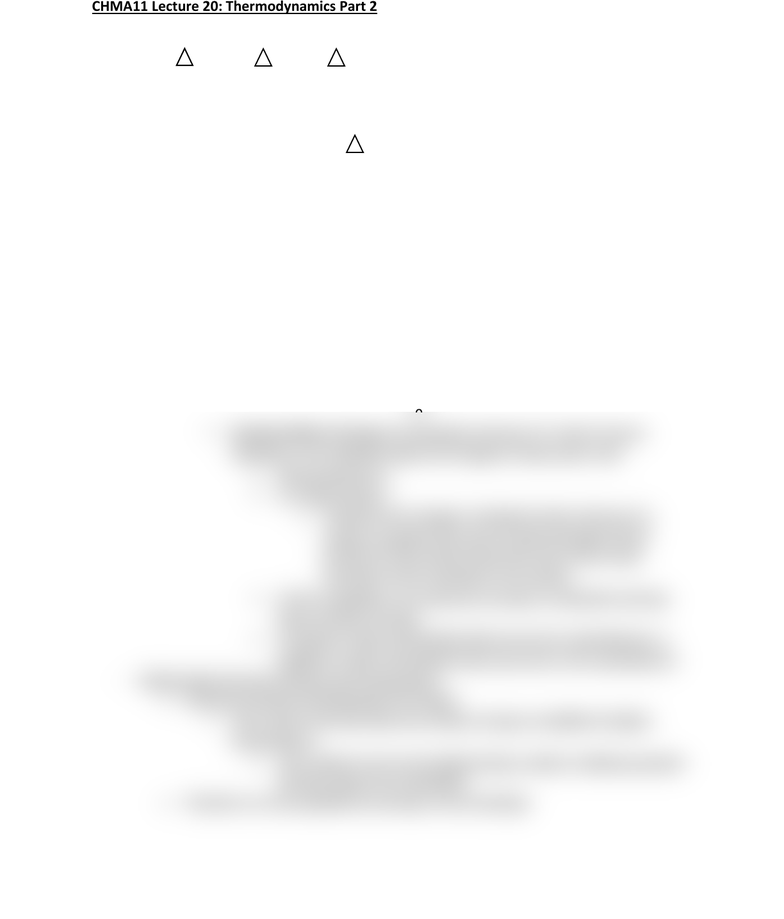# CHMA11H3 Lecture Notes - Lecture 23: Standard Molar Entropy, Zero Point Railway Station, Thermodynamics

66 views2 pages
School
Department
CourseCHMA11 Lecture 20: Thermodynamics Part 2
- Criteria for spontaneity
o Suniverse = Ssystem + Ssurroundings
Remember the 2nd law of thermodynamics
All spontaneous processes will produce increases in the entropy
of the universe
Therefore Suniverse will always be greater than 0
o Quantifying the entropy of systems
The absolute entropy of a substance can be measured
It is done in reference to a pure crystal of the substance in
question at a temperature of 0 K for which S will equal 0
o Zero entropy: the entropy of a perfect crystal at a 0 K is 0
This is because all motion will stop at a
temperature of 0 K
Zero Point Energy: tiny vibrations that molecules
exert on each other
Proof that there is still a little motion at 0 K
This energy is evenly distributed in a
perfectly crystal, hence the entropy value of
0
Standard Molar Entropy: the absolute entropy of 1 mole of a pure
substance in its standard state at 25 degrees Celsius and 1 atm
Represented by So
Is a state function
o Therefore the change in standard molar entropy of a
system is equal to the sum of molar entropies of the
products in the system minus the sum of the molar
entropies of the reactants in the system
As the complexity of a molecule increases, its absolute entropy
value will also increase
A positive S value will indicate that a process is spontaneous, a
negative S value will indicate that a process in non-spontaneous
- Relationship between Entropy and Temperature
o Entropy increases as temperature increases
This is due to the fact that more kinetic energy is available at higher
temperatures
This results in more microstates being created, enabling a greater
entropy value to be calculated
o Therefore we can quantify the entropy of surroundings
Unlock document

This preview shows half of the first page of the document.
Unlock all 2 pages and 3 million more documents.